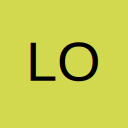# Index,Match Large

• I have used the Index, Match and Large function in a sheet to display the data for the top two items. It works fine when there is items with different values but doesn't work when there are two or more items with same highest values. How can I modify my formulae to achieve the result. I have attached the worksheet with comments in the formula.forum.ozgrid.com/index.php?attachment/55798/

## Files

• Re: Index,Match Large

Hi Pike, Thanks for the information. But I Have no problem finding the 1st and 2nd Largest. I mean the 1st and 2nd largest can be a same value, eg- 50 in my example. But my problem is how to trace the names from name item if the 1st and 2nd largest values are tied.

• Re: Index,Match Large

J4(drag to J5)
=INDEX(\$A\$4:\$A\$11,SMALL(IF(\$B\$4:\$B\$11=\$I4,ROW(\$B\$4:\$B\$11)-ROW(\$B\$4)+1),ROWS(\$A\$1:A1)))
Confirm Control+shift+Enter

• Re: Index,Match Large

you can use two fuctions which process the data indifferent ways
for the first value use

=VLOOKUP(I4,CHOOSE({1,2},B4:B11,A4:A11),2,0)

if look for the first true value
and for the second

=LOOKUP(2,1/(B4:B11=I5),A4:A11)

or

=INDEX(A\$4:A\$11,MATCH(I4,B\$4:B\$11))

they will return the last true value

• Re: Index,Match Large

just remembered
just use
=INDEX(A\$4:A\$11,MATCH(I4,B\$4:B\$11[COLOR="#FF0000"],-1[/COLOR]))
and
=INDEX(A\$4:A\$11,MATCH(I4,B\$4:B\$11[COLOR="#FF0000"],1[/COLOR]))

from the MATCH function help files

1 or omitted MATCH finds the largest value that is less than or equal to lookup_value. The values in the lookup_array argument must be placed in ascending order, for example: ...-2, -1, 0, 1, 2, ..., A-Z, FALSE, TRUE.
0 MATCH finds the first value that is exactly equal to lookup_value. The values in the lookup_array argument can be in any order.
-1 MATCH finds the smallest value that is greater than or equal to lookup_value. The values in the lookup_array argument must be placed in descending order, for example: TRUE, FALSE, Z-A, ...2, 1, 0, -1, -2, ..., and so on.

• Re: Index,Match Large

Hi Robert, The solution you provided is awesome. Thank you very much.

• Re: Index,Match Large

Quote from loknath;680235

Hi Robert, The solution you provided is awesome. Thank you very much.

You are welcome

## Participate now!

Don’t have an account yet? Register yourself now and be a part of our community!